# Lagrange multipliers

(diff) ← Older revision | Latest revision (diff) | Newer revision → (diff)
Jump to: navigation, search

Variables with the help of which one constructs a Lagrange function for investigating problems on conditional extrema. The use of Lagrange multipliers and a Lagrange function makes it possible to obtain in a uniform way necessary optimality conditions in problems on conditional extrema. The method of obtaining necessary conditions in the problem of determining an extremum of a function(1)

under the constraints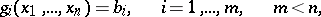(2)

consisting of the use of Lagrange multipliers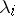,, the construction of the Lagrange function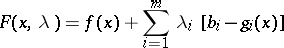and equating its partial derivatives with respect to the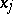andto zero, is called the Lagrange method. In this method the optimal valueis found together with the vector of Lagrange multipliers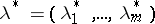corresponding to it by solving the system of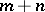equations. The Lagrange multipliers,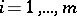, have the following interpretation . Suppose that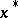provides a relative extremum of the function (1) under the constraints (2):. The values of,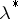anddepend on the values of the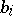, the right-hand sides of the constraints (2). One has formulated quite general assumptions under which all the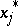and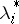are continuously-differentiable functions of the vector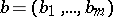in some-neighbourhood of its value specified in (2). Under these assumptions the functionis also continuously differentiable with respect to the. The partial derivatives of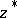with respect to theare equal to the corresponding Lagrange multipliers, calculated for the given: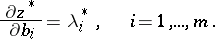(3)

In applied problemsis often interpreted as profit or cost, and the right-hand sides,, as losses of certain resources. Then the absolute value ofis the ratio of the unit cost to the unit-th resource. The numbersshow how the maximum profit (or maximum cost) changes if the amount of the-th resource is increased by one. This interpretation of Lagrange multipliers can be extended to the case of constraints in the form of inequalities and to the case when the variablesare subject to the requirement of being non-negative.

In the calculus of variations one conveniently obtains by means of Lagrange multipliers necessary conditions for optimality in the problem on a conditional extremum as necessary conditions for an unconditional extremum of a certain composite functional. Lagrange multipliers in the calculus of variations are not constants, but certain functions. In the theory of optimal control and in the Pontryagin maximum principle, Lagrange multipliers have been called conjugate variables.